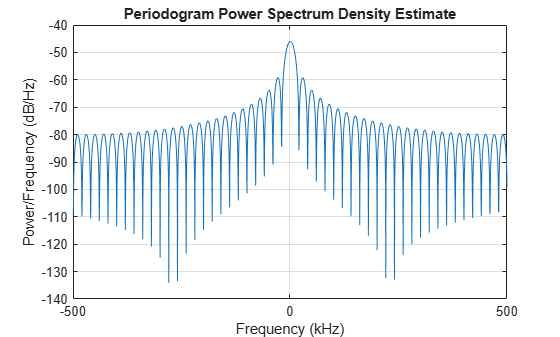# step

System object: phased.StretchProcessor
Package: phased

Perform stretch processing for linear FM waveform

## Syntax

```Y = step(H,X) Y = step(H,X,PRF) ```

## Description

Note

Starting in R2016b, instead of using the `step` method to perform the operation defined by the System object™, you can call the object with arguments, as if it were a function. For example, ```y = step(obj,x)``` and `y = obj(x)` perform equivalent operations.

`Y = step(H,X)` applies stretch processing along the first dimension of `X`. Each column of `X` represents one receiving pulse.

`Y = step(H,X,PRF)` uses `PRF` as the pulse repetition frequency. This syntax is available when the `PRFSource` property is ```'Input port'```.

## Input Arguments

 `H` Stretch processor object. `X` Input signal matrix. Each column represents one received pulse.The size of the first dimension of the input matrix can vary to simulate a changing signal length. A size change can occur, for example, in the case of a pulse waveform with variable pulse repetition frequency. `PRF` Pulse repetition frequency specified as a positive scalar. To enable this argument, set the `PRFSource` property to ```'Input port'```. Units are in Hertz.

## Output Arguments

 `Y` Result of stretch processing. The dimensions of `Y` match the dimensions of `X`.

## Examples

expand all

Use stretch processing to locate a target at a range of 4950 m.

Note: This example runs only in R2016b or later. If you are using an earlier release, replace each call to the function with the equivalent `step` syntax. For example, replace `myObject(x)` with `step(myObject,x)`.

Simulate the signal.

```waveform = phased.LinearFMWaveform; x = waveform(); c = physconst('LightSpeed'); rng = 4950.0; num_samples = round(rng/(c/(2*waveform.SampleRate))); x = circshift(x,num_samples);```

Perform stretch processing.

```stretchproc = getStretchProcessor(waveform,5000,200,c); y = stretchproc(x);```

Plot the spectrum of the resulting signal.

```[Pxx,F] = periodogram(y,[],2048,stretchproc.SampleRate,'centered'); plot(F/1000,10*log10(Pxx)) grid xlabel('Frequency (kHz)') ylabel('Power/Frequency (dB/Hz)') title('Periodogram Power Spectrum Density Estimate')```Detect the range.

```[~,rngidx] = findpeaks(pow2db(Pxx/max(Pxx)),'MinPeakHeight',-5); rngfreq = F(rngidx); rng = stretchfreq2rng(rngfreq,stretchproc.SweepSlope,stretchproc.ReferenceRange,c)```
```rng = 4.9634e+03 ```